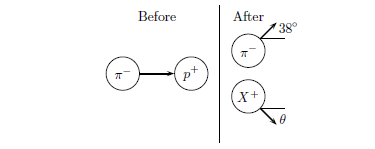# Problem: A beam of high-energy π− (negative pions) is shot at a flask of liquid hydrogen, and sometimes a pion interacts through the strong interaction with a proton in the hydrogen, in the reaction π− + p+ → π− + X+, where X+ is a positively charged particle of unknown mass. The incoming pion momentum is 2965 MeV/c. The pion is scattered through 38°, and its momentum is measured to be 1480 MeV/c. The X+ particle is scattered through an unknown angle θ with an unknown momentum. A pion has a rest energy of 140 MeV, and a proton has a rest energy of 938 MeV. Find the scattering angle θ of the X+ particle. 1. 25.8446 2. 29.2199 3. 29.9574 4. 24.9877 5. 25.4691 6. 27.5308 7. 28.7532 8. 26.8651 9. 28.454 10. 27.815

###### FREE Expert Solution
87% (227 ratings)
###### Problem Details

A beam of high-energy π (negative pions) is shot at a flask of liquid hydrogen, and sometimes a pion interacts through the strong interaction with a proton in the hydrogen, in the reaction π + p+ → π + X+, where X+ is a positively charged particle of unknown mass. The incoming pion momentum is 2965 MeV/c. The pion is scattered through 38°, and its momentum is measured to be 1480 MeV/c. The X+ particle is scattered through an unknown angle θ with an unknown momentum. A pion has a rest energy of 140 MeV, and a proton has a rest energy of 938 MeV. Find the scattering angle θ of the X+ particle.

1. 25.8446
2. 29.2199
3. 29.9574
4. 24.9877
5. 25.4691
6. 27.5308
7. 28.7532
8. 26.8651
9. 28.454
10. 27.815